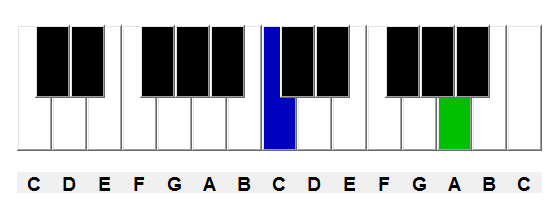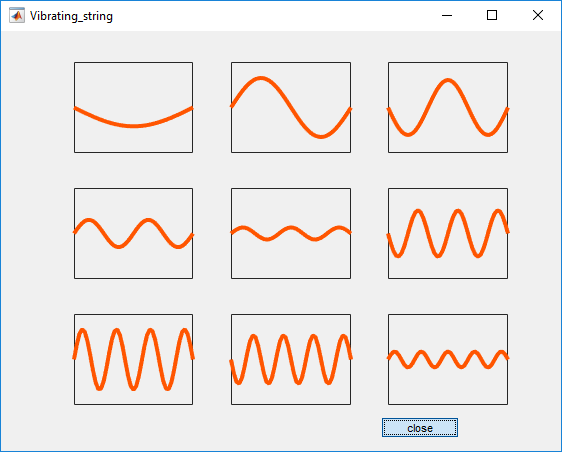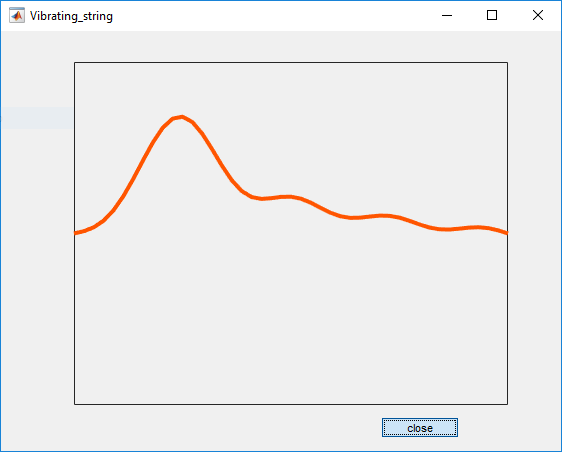# Math and Music

What does $\sqrt{2}$ have to do with music? What are equal temperament and just intonation? How can the MATLAB function rats help tune a piano? (This post is based in part on the Music chapter in my online book, Experiments in MATLAB.)

### Contents

#### Octaves and semitones

In the theory of music, an octave is an interval with frequencies that range over a factor of two. In most Western music, an octave is divided into 12 semitones in a geometric progression. In other words the notes have equal frequency ratios. Since 12 semitones comprise a factor of 2, a single semitone is a factor of $\sqrt{2}$. And because this quantity occurs so often in this discussion, let

$$\sigma = \sqrt{2}$$

Our MATLAB programs use

    format short
sigma = 2^(1/12)

sigma =
1.0595


Think of $\sigma$ as an important mathematical constant, like $\pi$ and $\phi$.

#### Keyboard

Here is our miniature piano keyboard with 25 keys.

     small_keyboardThis keyboard has two octaves, with white keys labeled C D … G A B, plus another C key. Counting both white and black, there are twelves keys in each octave. The frequency of each key is a semitone above or below its neighbors. Each black key can be regarded as either the sharp of the white below it or the flat of the white above it. So the black key between C and D is both C $\sharp$ and D $\flat$. There are no E $\sharp$ / F $\flat$ or B $\sharp$ / C $\flat$.

A conventional full piano keyboard has 88 keys. Seven complete octaves account for $7 \times 12 = 84$ keys. There are three additional keys at the lower left and one additional key at the upper end. If the octaves are numbered 0 through 7, then a key letter followed by an octave number specifies a unique key. In this notation, two important keys are C4 and A4. The C4 key is near the center of the keyboard and so is also known as middle C. A piano is usually tuned so that the frequency of the A4 key is 440 Hz. C4 is nine keys to the left of A4 so its frequency is

$$\mbox{C4} = 440 \sigma^{-9} \approx 261.6256 \mbox{Hz}$$

Our miniature keyboard has the A4 key colored green and the C4 key colored blue.

#### Equal Temperament

A piano is almost always tuned with equal temperament, the system used by musical instruments with fixed pitch. Starting with A4 at 440 Hz the keys are tuned in strict geometric progression with ratio $\sigma$. Once this is done, a piano rarely needs adjustment.

#### Just Intonation

The alternative system, just intonation, is defined by a vector of ratios involving small integers.

   format rat
just = [1 16/15 9/8 6/5 5/4 4/3 7/5 3/2 8/5 5/3 7/4 15/8 2]'

just =
1
16/15
9/8
6/5
5/4
4/3
7/5
3/2
8/5
5/3
7/4
15/8
2


These ratios allow the generation of overtones, chords and harmony. More about that in a moment. Many musical instruments can be tuned to the key of a particular composition just before playing it. Singers, choirs, and barbershop quartets can naturally use just intonation.

Here is a comparison of equal temperament and just intonation from a strictly numerical point of view. Equal temperament is defined by repeated powers of $\sigma$. while just intonation is defined by a sequence of ratios.

   sigma = 2^(1/12);
k = (0:12)';
equal = sigma.^k;
num = [1 16 9 6 5 4 7 3 8 5 7 15 2]';
den = [1 15 8 5 4 3 5 2 5 3 4  8 1]';
just = num./den;
delta = (equal - just)./equal;
T = [k equal num den just delta];
fprintf('   k       equal           just              delta\n')
fprintf('%4d %12.6f %7d/%d %11.6f %10.4f\n',T')

   k       equal           just              delta
0     1.000000       1/1    1.000000     0.0000
1     1.059463      16/15    1.066667    -0.0068
2     1.122462       9/8    1.125000    -0.0023
3     1.189207       6/5    1.200000    -0.0091
4     1.259921       5/4    1.250000     0.0079
5     1.334840       4/3    1.333333     0.0011
6     1.414214       7/5    1.400000     0.0101
7     1.498307       3/2    1.500000    -0.0011
8     1.587401       8/5    1.600000    -0.0079
9     1.681793       5/3    1.666667     0.0090
10     1.781797       7/4    1.750000     0.0178
11     1.887749      15/8    1.875000     0.0068
12     2.000000       2/1    2.000000     0.0000


The last column in the table, delta, is the relative difference between the two. We see that delta is less than one percent, except for k = 10.

This is why the 12-note scale has proved to be so popular. Powers of $\sqrt{2}$ turn out to come very close to important rational numbers, especially 5/4, 4/3, and 3/2. A pair of pitches with a ratio of 3:2 is known as a perfect fifth; a ratio of 4:3 is a perfect fourth; a ratio of 5:4 is a major third.

#### Do Re Mi

One of the first songs you learned to sing was

Do Re Mi Fa So La Ti Do

If you start at middle C, you will be singing the major scale in the key of C. This scale is played on a piano using only the white keys. The steps are not equally spaced. Most of the steps skip over black keys and so are two semitones. But the steps between Mi and Fa and Ti and Do are the steps from E to F and B to C. There are no intervening black keys and so these steps are only one semitone. In terms of $\sigma$, the C-major scale is

$$\sigma^0 \ \sigma^2 \ \sigma^4 \ \sigma^5 \ \sigma^7 \ \sigma^9 \ \sigma^{11} \ \sigma^{12}$$

The number of semitones between the notes is given by the vector

    format short
diff([0 2 4 5 7 9 11 12])

ans =
2     2     1     2     2     2     1


This sequence of frequencies in our most common scale is surprising.

#### Vibrations and Waves

Like everything else, it all comes down to eigenvalues. Musical instruments create sound through the action of vibrating strings or vibrating columns of air that, in turn, produce vibrations in the body of the instrument and the surrounding air. Mathematically, vibrations can be modeled by weighted sums of characteristic functions known as modes or eigenfunctions. Different modes vibrate at different characteristic frequencies or eigenvalues. These frequencies are determined by physical parameters such as the length, thickness and tension in a string, or the geometry of the air cavity. Short, thin, tightly stretched strings have high frequencies, while long, thick, loosely stretched strings have low frequencies.

The simplest model is a one-dimensional vibrating string, held fixed at its ends. The units of the various physical parameters can be chosen so that the length of the string is $2 \pi$. The modes are then simply the functions

$$v_n(x) = \sin{n x}, \ \, n = 1, 2, …$$

Each of these functions satisfy the fixed end point conditions

$$v_n(0) = v_n(2 \pi) = 0$$

The time-dependent modal vibrations are

$$u_n(x,t) = \sin{n x} \sin{n t}, \ \, n = 1, 2, …$$

and the frequency is simply the integer $n$. (Two- and three-dimensional models are much more complicated. This one-dimensional model is all we need here.)

Our Experiments with MATLAB program vibrating_string provides a dynamic view. Here is a snapshot showing the first nine modes and the resulting wave traveling along the string.The ratios in just intonation or equal temperament produce instruments with tunings that generate vibrations with these desired frequencies.

#### rat and rats

The MATLAB expression rat(X,tol) creates a truncated continued fraction approximation of X with an accuracy of tol. The default tol is 1.e-6*norm(X,1).

   rat(sigma.^(0:12)')

ans =
1
1 + 1/(17 + 1/(-5 + 1/(-2)))
1 + 1/(8 + 1/(6))
1 + 1/(5 + 1/(4 + 1/(-2)))
1 + 1/(4 + 1/(-7 + 1/(2 + 1/(5))))
1 + 1/(3 + 1/(-74))
1 + 1/(2 + 1/(2 + 1/(2 + 1/(2 + 1/(2 + 1/(2))))))
1 + 1/(2 + 1/(147))
2 + 1/(-2 + 1/(-2 + 1/(-3 + 1/(4 + 1/(2)))))
2 + 1/(-3 + 1/(-7 + 1/(-81)))
2 + 1/(-5 + 1/(2 + 1/(3 + 1/(-2 + 1/(-15)))))
2 + 1/(-9 + 1/(11))
2


These are unconventional continued fractions because they contain negative terms. This algorithm is used for format rat. The default tolerance is much too picky. The equal temperament ratios do not give small integers.

   format rat
sigma.^(0:12)'

ans =
1
1657/1564
1769/1576
1785/1501
635/504
3544/2655
1393/985
2655/1772
1008/635
3002/1785
1527/857
2943/1559
2


This would not be a satisfactory way to try to tune an instrument. But if I’m much more tolerant and allow a 2 percent error, I get short continued fractions.

   rat(sigma.^(0:12)',.02)

ans =
1
1 + 1/(17)
1 + 1/(8)
1 + 1/(5)
1 + 1/(4)
1 + 1/(3)
1 + 1/(2 + 1/(2))
1 + 1/(2)
2 + 1/(-2 + 1/(-2))
2 + 1/(-3)
2 + 1/(-5)
2 + 1/(-9)
2


I can make format rat use these fractions by telling rats that it has only 6 columns to work with. Now I get almost the same ratios as just intonation.

   rats(sigma.^(0:12)',6)

ans =
1
1
9/8
6/5
5/4
4/3
7/5
3/2
8/5
5/3
9/5
17/9
2


#### Rewriting Music History

If any of the music theorists from ancient Greece or the Renaissance had access to MATLAB, they could have used our rational approximation to generate a tuning strategy.

Published with MATLAB® R2016a

|

### コメント

コメントを残すには、ここ をクリックして MathWorks アカウントにサインインするか新しい MathWorks アカウントを作成します。